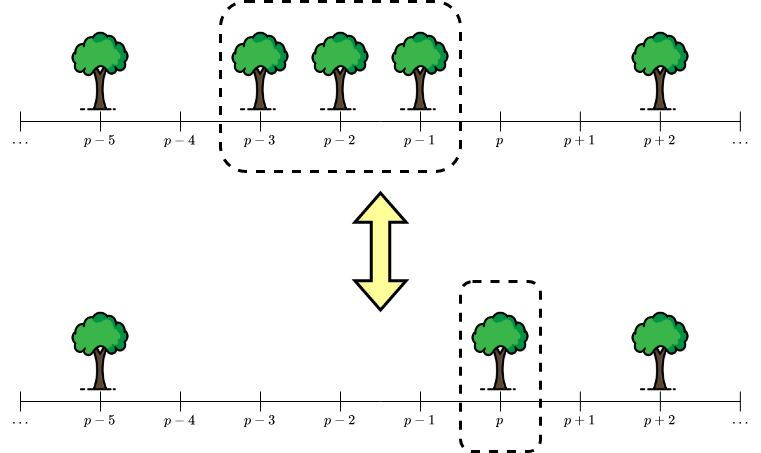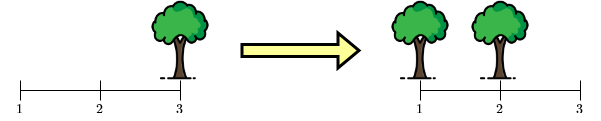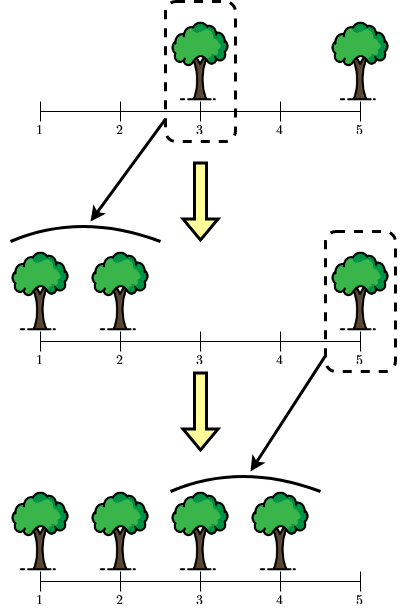## The forest gods' apprenticeship

View as PDF

Points: 1.20 (partial)
Time limit: 2.0s
Memory limit: 256M
Input: stdin
Output: stdout

Problem type
Allowed languages
C, C++, Java, Kotlin, Pascal, PyPy, Python, Scratch
Một cây làm chẳng nên non. ~k~ cây chụm lại nên hòn núi cao
Literal translation: One tree cannot amount to anything, ~k~ of them together can look like a mountain.

Every forest deity have a forest to manage. Each forest has ~n~ positions, numbered from ~1~ to ~n~, and each position has at most one tree.

It is well known that a forest god with power level ~k~ can perform the following action on his forest:

• If there are no trees in position ~p~ (~k < p \le n~), but there is a tree in each positions ~p - 1, p - 2, \ldots, p - k~, the deity can cast a magic spell to merge these ~k~ trees to make a new one at position ~p~. After casting the magic spell, trees at positions ~p - 1, p - 2, \ldots, p - k~ will disappear, and a new tree appears at position ~p~.

• If there currently exists a tree at position ~p~ (~k < p \le n~), and there are no trees at positions ~p - 1, p - 2, \ldots, p - k~, the deity can cast a magic spell to plant ~k~ trees at the aforementioned positions. After casting the magic spell, the tree at position ~p~ will disappear, and a new tree will appear at each of the positions ~p - 1, p - 2, \ldots, p - k~ .Illustration for a spell casting for ~k = 3~.

There are two apprentice forest deities with power level ~k~, Quang and Tùng. Quang manages the forest Q, where as Tùng is in charge of the forest T. Quang is a hard-working god, practicing his spell every day to perfect his skill. Tùng is a polar opposite. A lazy cat he is, he likes to have fun climbing the trees in his forest (and would frequently forget his way down and get stuck on a tree afterwards). Due to this, he does not practice his spells frequently.

Eventually after all the practicing, it pays off, Quang has cultivated his forest Q to be evergreen and beautiful. Tùng's forest T however, was hardly looked after, and thus was dark and gloomy. Of course Tùng was jealous of Quang of this. But despite that, he's not going to make a scene of it, as he's well aware that it was his own fault. He decided to make a change and to actually work to make his forest better this time.

Given the power level of the two deities and the two forests' layout. Help the forest god Tùng answer ~q~ questions:

• Given three integers ~l~, ~x~ and ~y~. Consider these two forests:

• The forest X created by taking the ~l~ consecutive positions of T starting from position ~x~,

• The forest Y created by taking the ~l~ consecutive positions of Q starting from position ~y~.

Is it possible to transform X into Y using the spell several (possibly zero) times?

Note that X and Y are two forests by their own. These two forests both have ~l~ positions, numbered from ~1~ to ~l~. They both do not have position ~l + 1~, thus Tùng cannot create a tree at this position.

Forest X and Y are considered to be the same, iff there does not exist a position, such that there is a tree in one forest, but not in the other.

#### Input

The first line contains three integers ~n~, ~k~, and ~q~ (~k < n \le 10^5~, ~1 \le k \le 5~, ~1 \le q \le 10^5~), denoting the length of a forest, the power of level of the two forest deities, and the number of questions in Tùng's mind.

The next line contains a binary string ~T~ of length ~n~, describing the forest T. There is a tree in position ~i~ of forest T iff ~T_i = 1~.

The next line contains a binary string ~Q~ of length ~n~, describing the forest Q. There is a tree in position ~i~ of forest Q iff ~Q_i = 1~.

The next ~q~ lines, each contains three integers ~l~, ~x~, and ~y~ (~k < l \le n~, ~1 \le x, y \le n - l + 1~), denoting a question.

#### Output

For each question, output YES if it's possible to transform forest X (by taking ~l~ positions in T starting from ~x~) into forest Y (by taking ~l~ positions in Q starting from ~y~). Otherwise, output NO.

#### Scoring

• Subtask 1 (~95~ points): ~n, q \le 3000~

The total score for this problem is ~310~.

#### Sample Input 1

8 2 4
00101001
11011110
3 3 2
3 1 6
5 1 4
4 2 3


#### Sample Output 1

YES
YES
YES
NO


#### Notes

In the first question, both forest are already the same (they both are 101).

In the second question, forest X has the form 001, and forest Y has the form 110. Tùng can transform the tree at position ~3~ inX into two trees at positions ~1~ and ~2~.Illustration of the second question.

In the third question, forest X has the form 00101, and forest Y has the form 11110. We can perform operations as follow:Illustration of the third question. Tùng can first transform the tree at position ~3~ inX into two trees at positions ~1~ and ~2~, then transform the tree at position ~5~ into two trees at positions ~3~ and ~4~.

In the last question, forest X has the form 0101, and forest Y has the form 0111. It can be shown that it is impossible to turn X into forest Y.